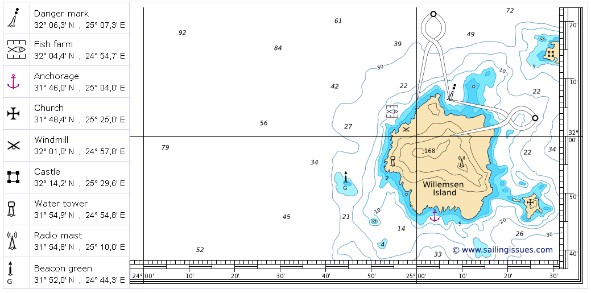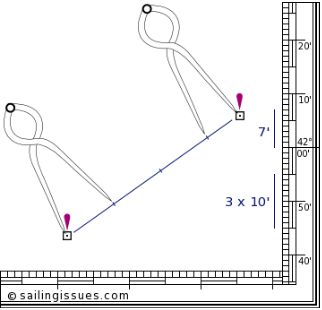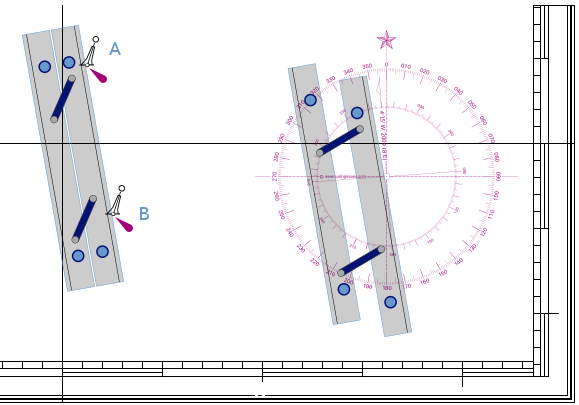## Coordinates and positions

A pair of nautical dividers (single handed dividers) is used to obtain precise coordinates from the chart. This device enables you to take the distance between that particular position and the closest grid line. You then place the dividers on the scale with one end on this grid line, leaving the other end precisely at your coordinate. Do this twice to get both latitude and longitude at the scale on the edge of the chart.
Below are some examples.DISTANCES

To measure the distance between, for instance, these two oil rigs, we will again need our dividers. Remember, we can only use the vertical scale. We first take a convenient distance like 10' (10 nautical miles) on the vertical scale using the middle latitude. Then we start walking with the dividers from the southern oil rig to northern one. Finally, we adjust the dividers to measure the small remaining part at its own height, i.e. its own latitude.
The image shows that the total distance is 37 nautical milesCOURSES

So, now we can measure distances and both plot and read out positions, but we also need directions. For example we need to find the course from safe-water buoy A to safe-water buoy B. To accomplish this we may use parallel rules as shown in this chart :

First you line this instrument up with the two buoys.

Then follows the intriguing part in moving the device to the compass rose without losing its alignment.

Finally, when one of the rules is aligned with the heart of the compass card, you can read course AB. In this example: 170°.
Besides the parallel rules there are other types of instruments available.